# Selina Solutions for Class 9 Physics Chapter 8 - Propagation of Sound waves

Selina solutions for class 9 Chapter 8 Propagation of Sound waves provides complete information regarding sound waves and transmission of these waves through a medium. ICSE Class 9 students can refer to these solutions for this chapter and all the chapters as the solutions have been constructed with the information provided in the ICSE textbook in accordance to the C.IS.C.E.

Students get overwhelmed with the thought of examinations often and in a hurry to tick all the checkboxes when it comes to exam preparation, tend to forget what they had studied. Selina solutions serve as a single-window for all the academic requirements of class 9 students.

Solutions are designed in accordance with the latest syllabus prescribed by the board. Use of a simple language and easy to understand terminologies makes ICSE Class 9 Physics Selina solutions students’ favourite and hence perform exceedingly well at the examinations.

### Access Answers of Physics Selina class 9 Chapter 8 – Propagation of Sound waves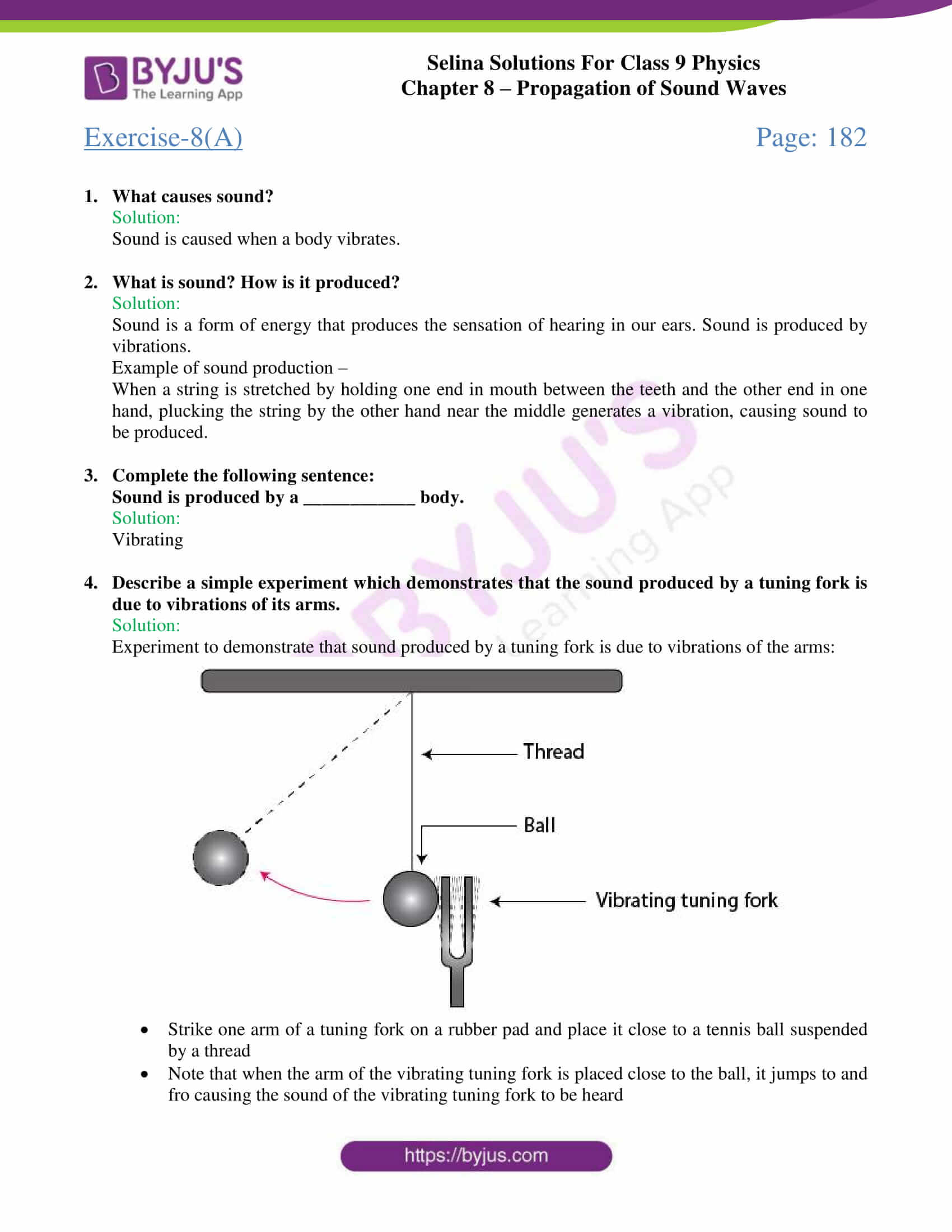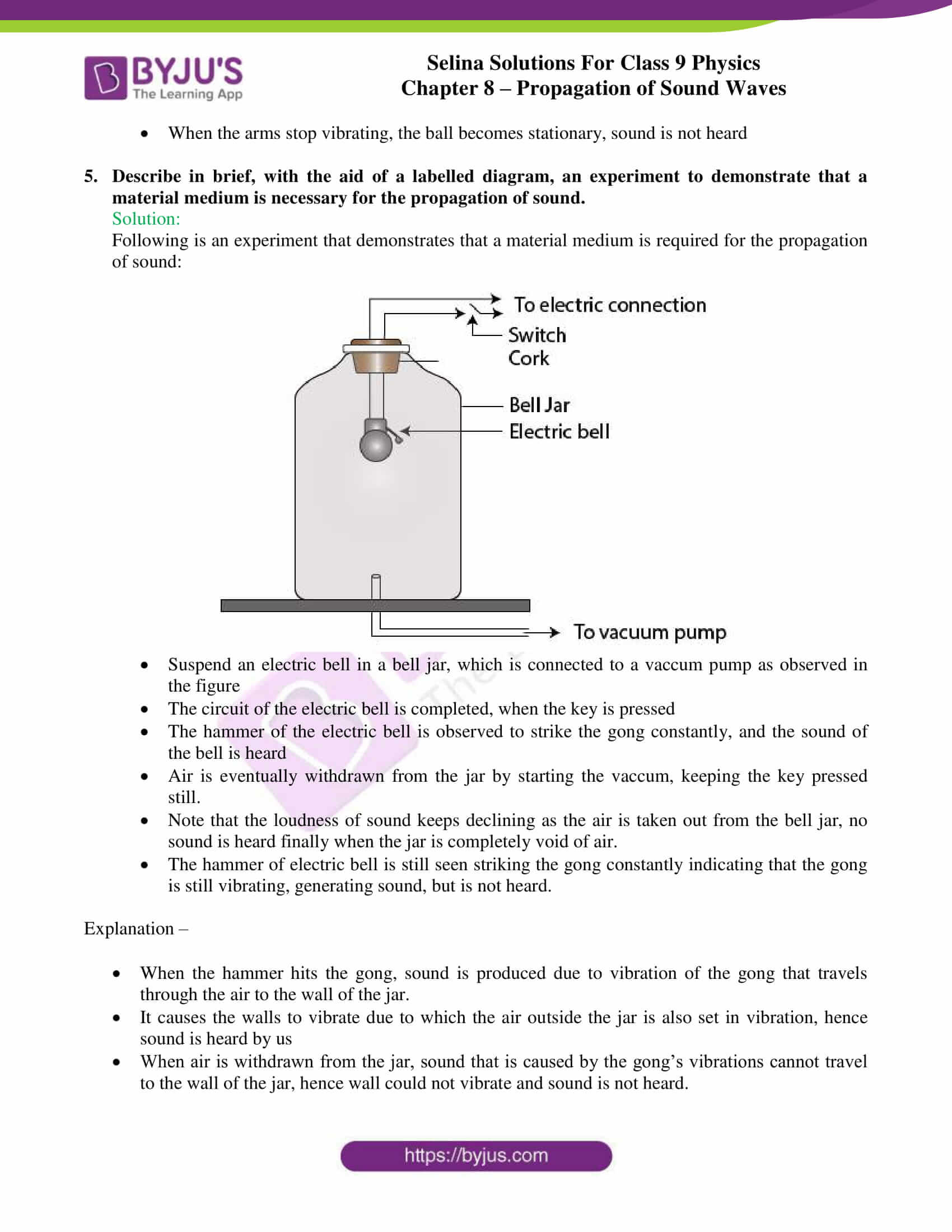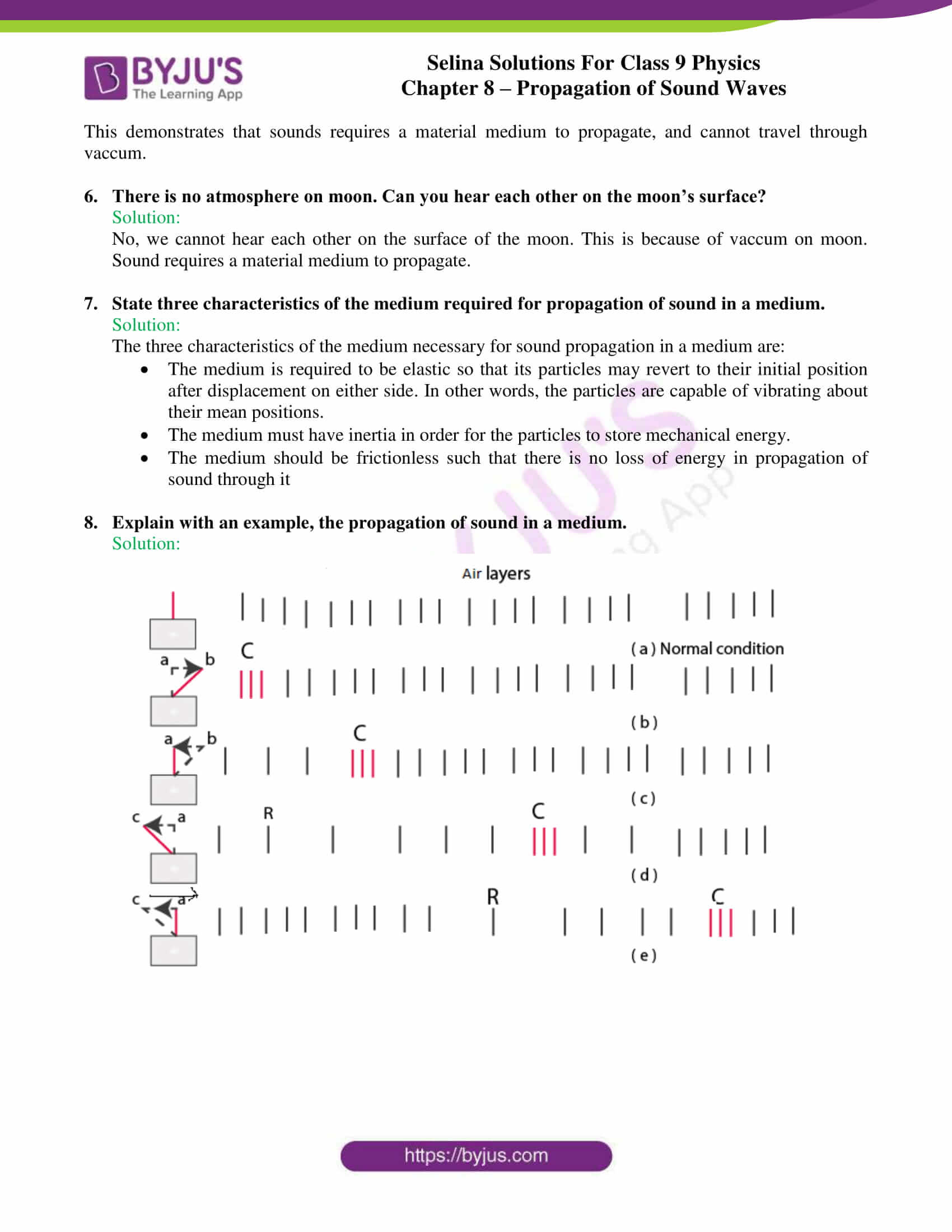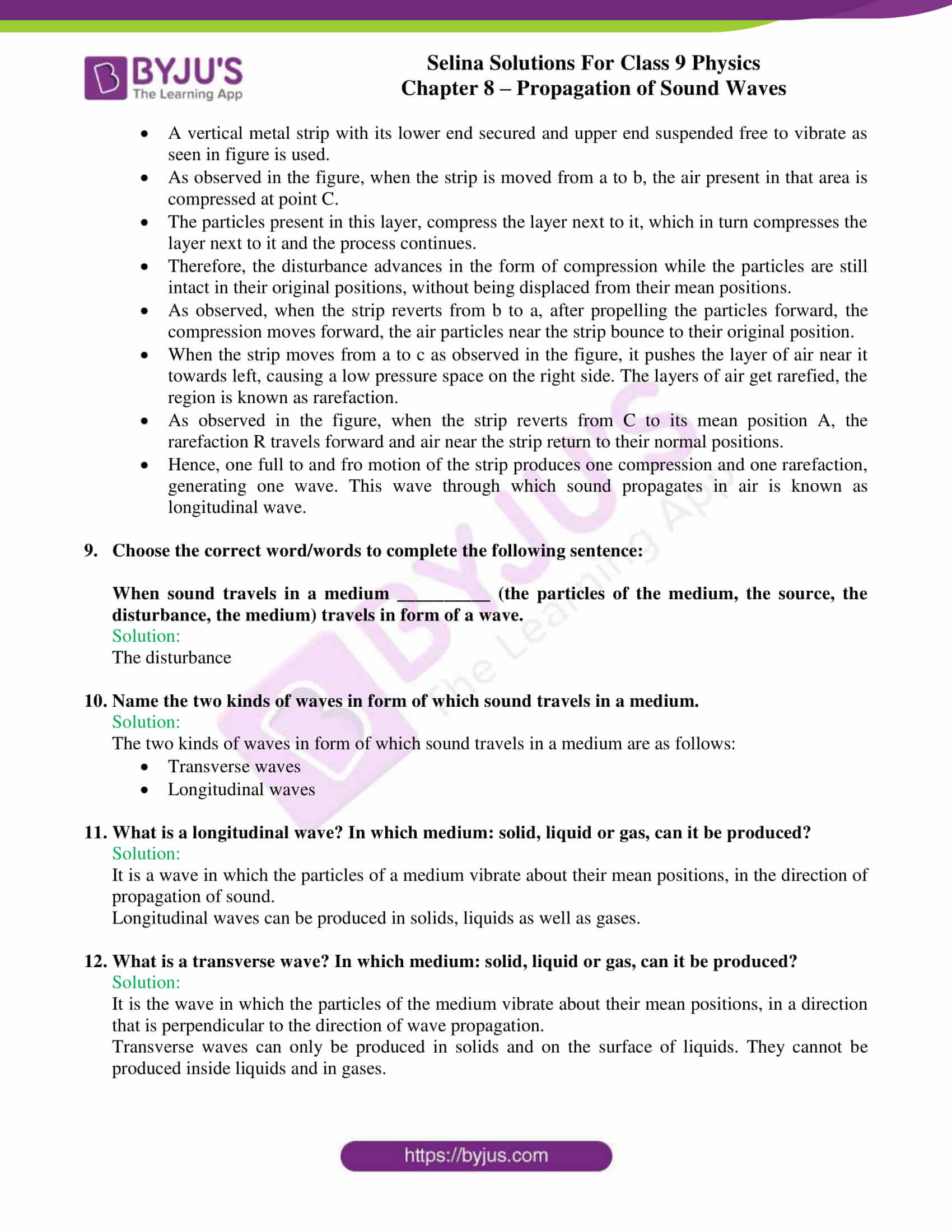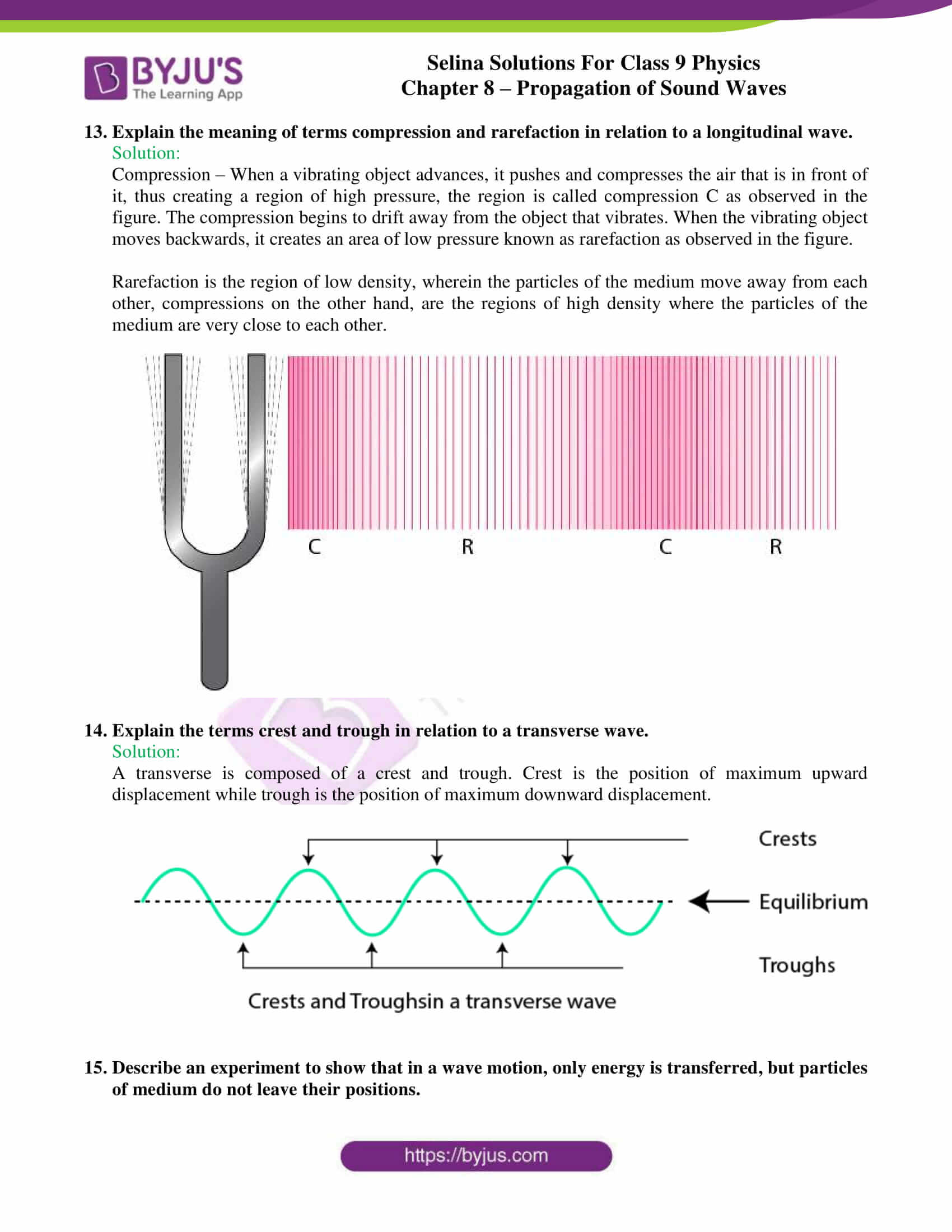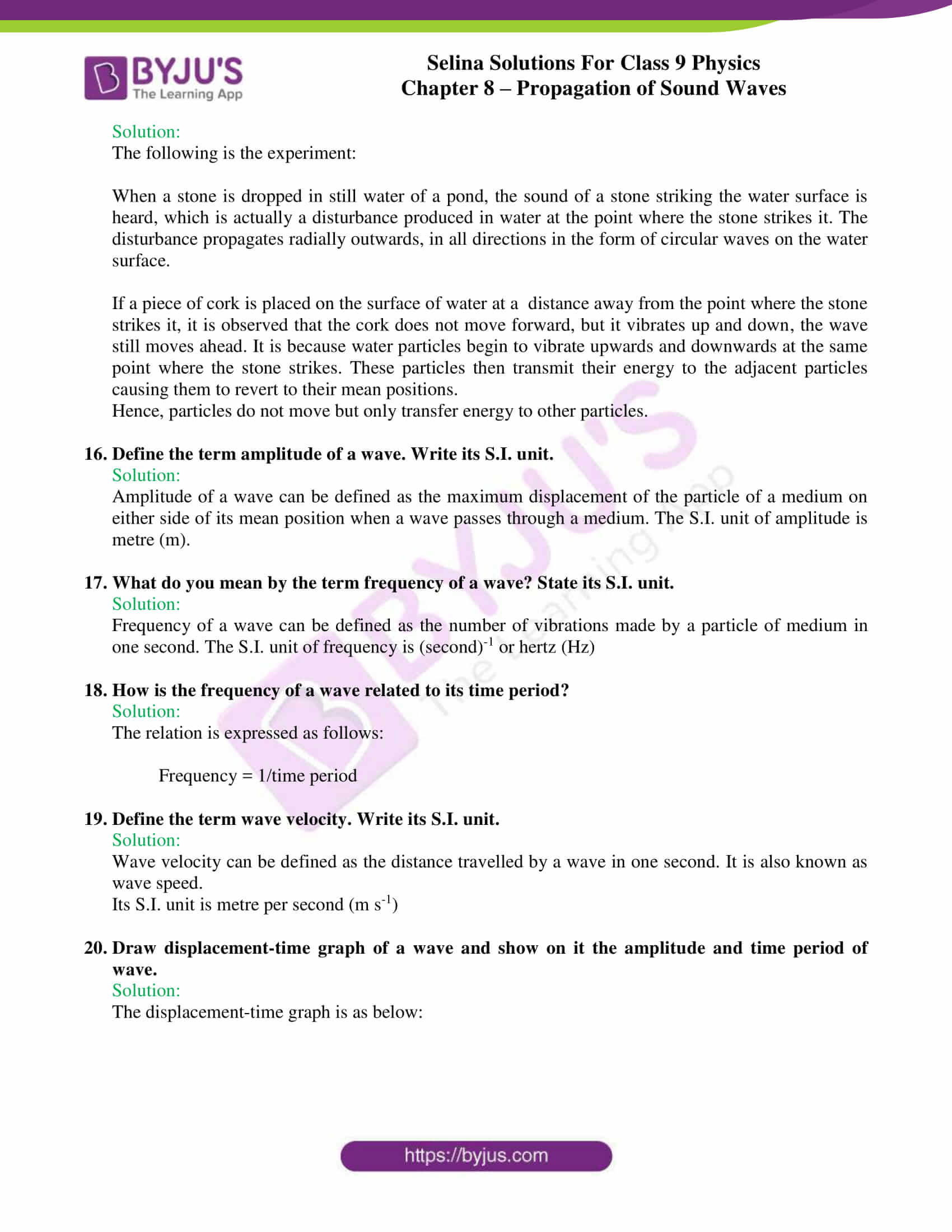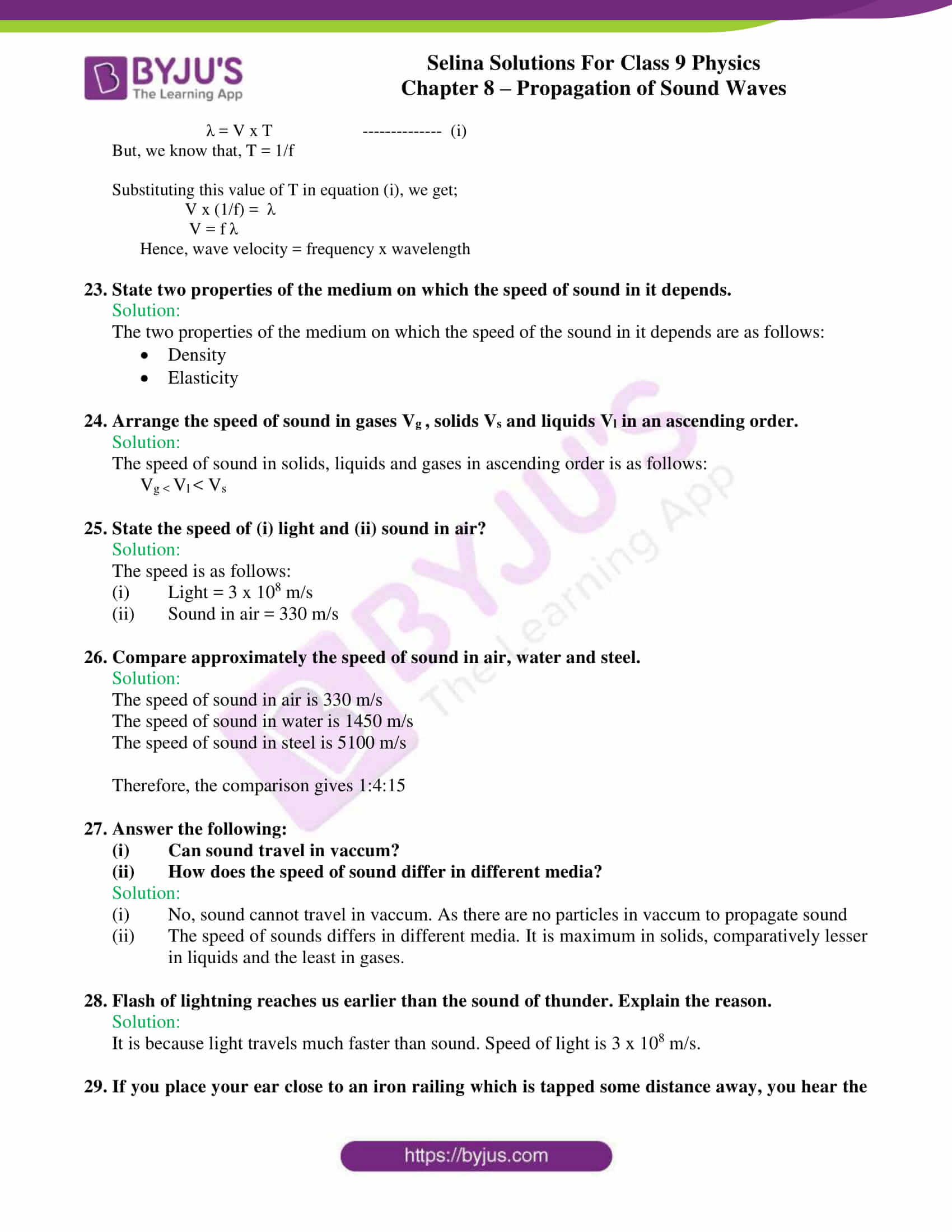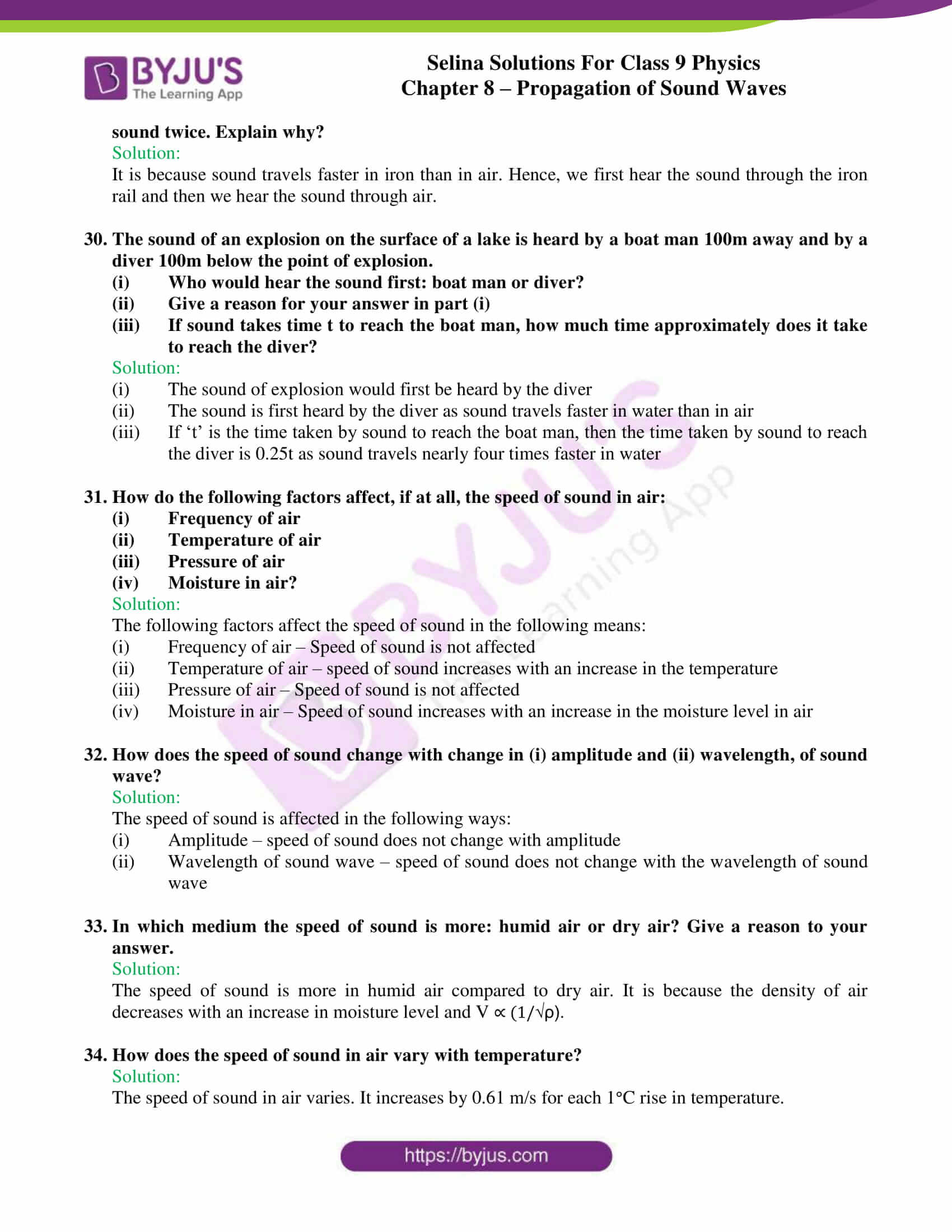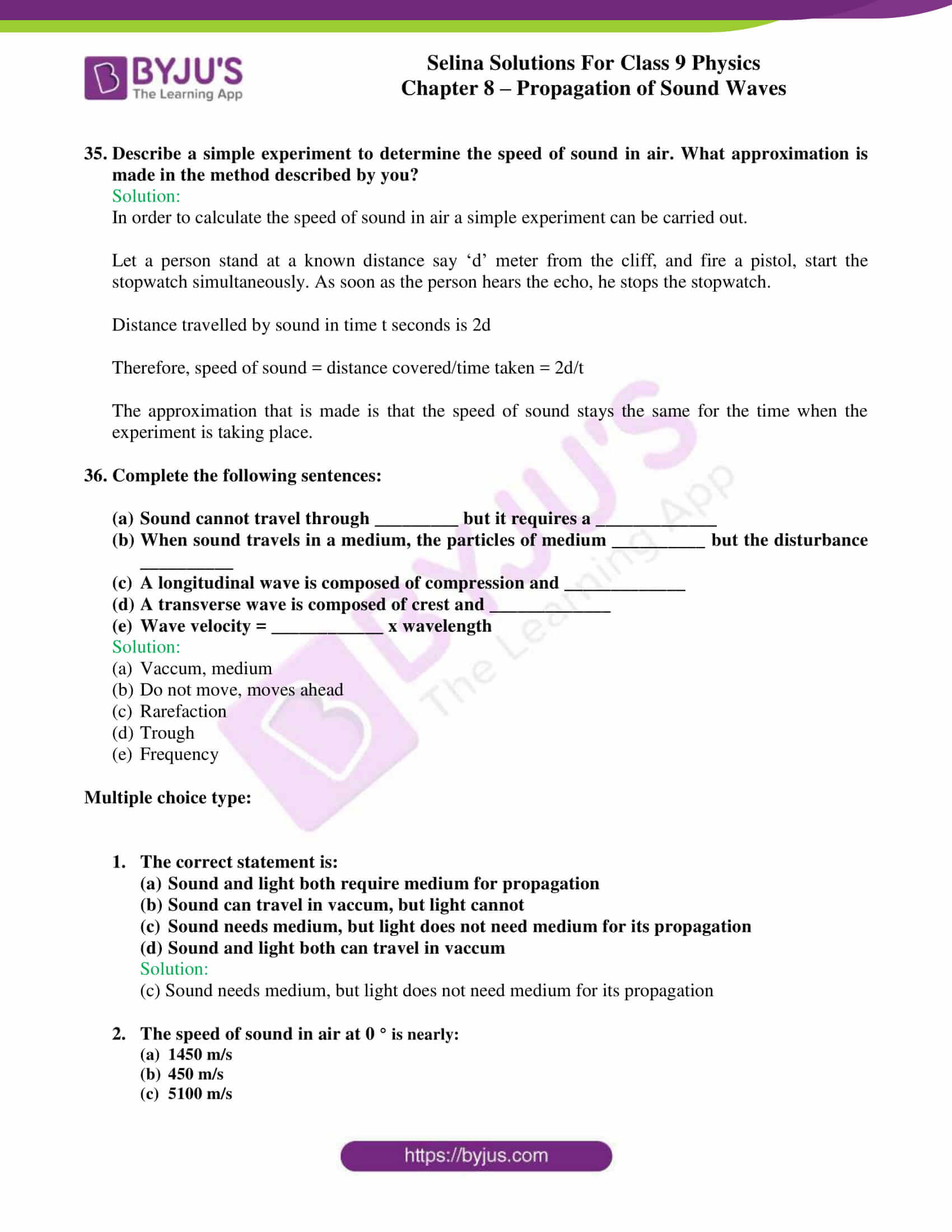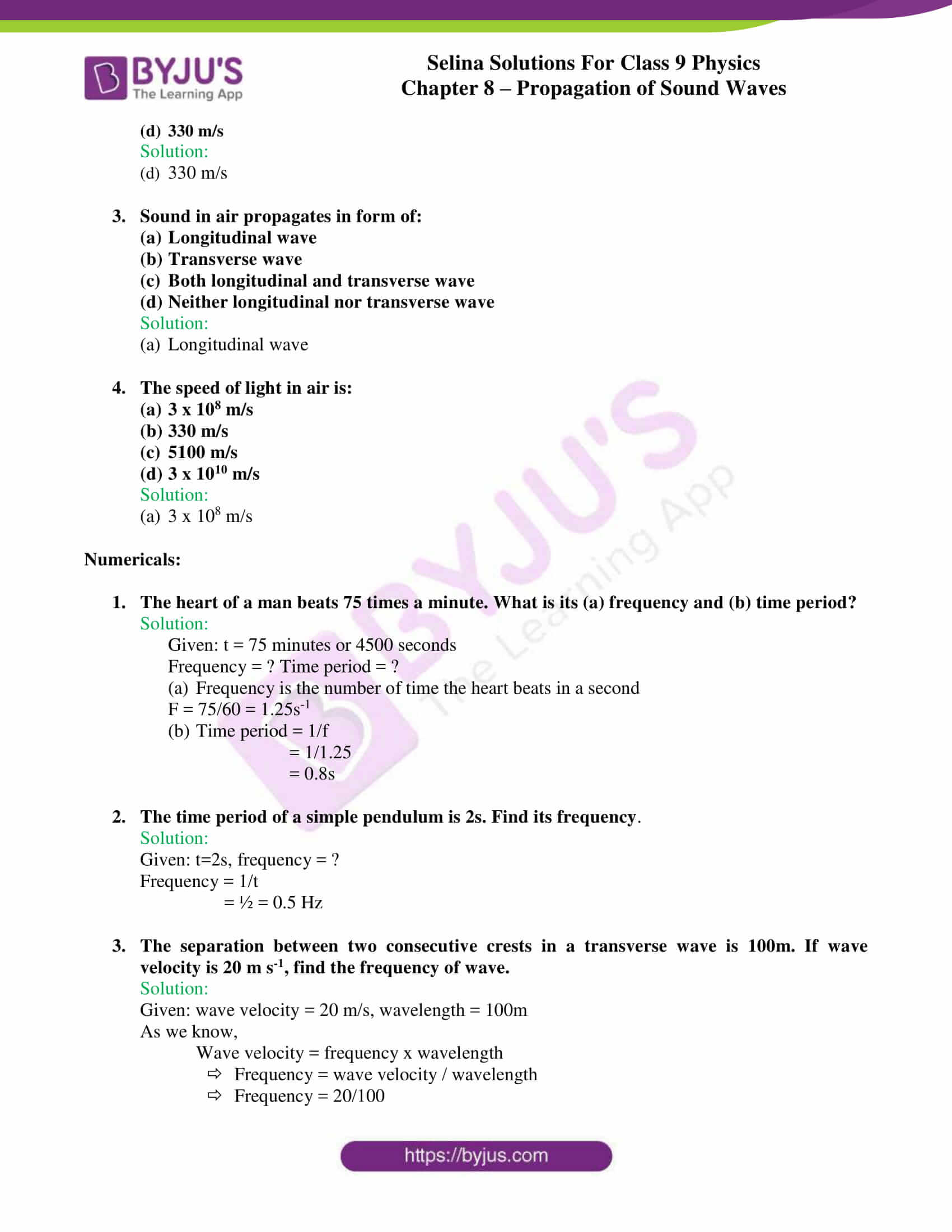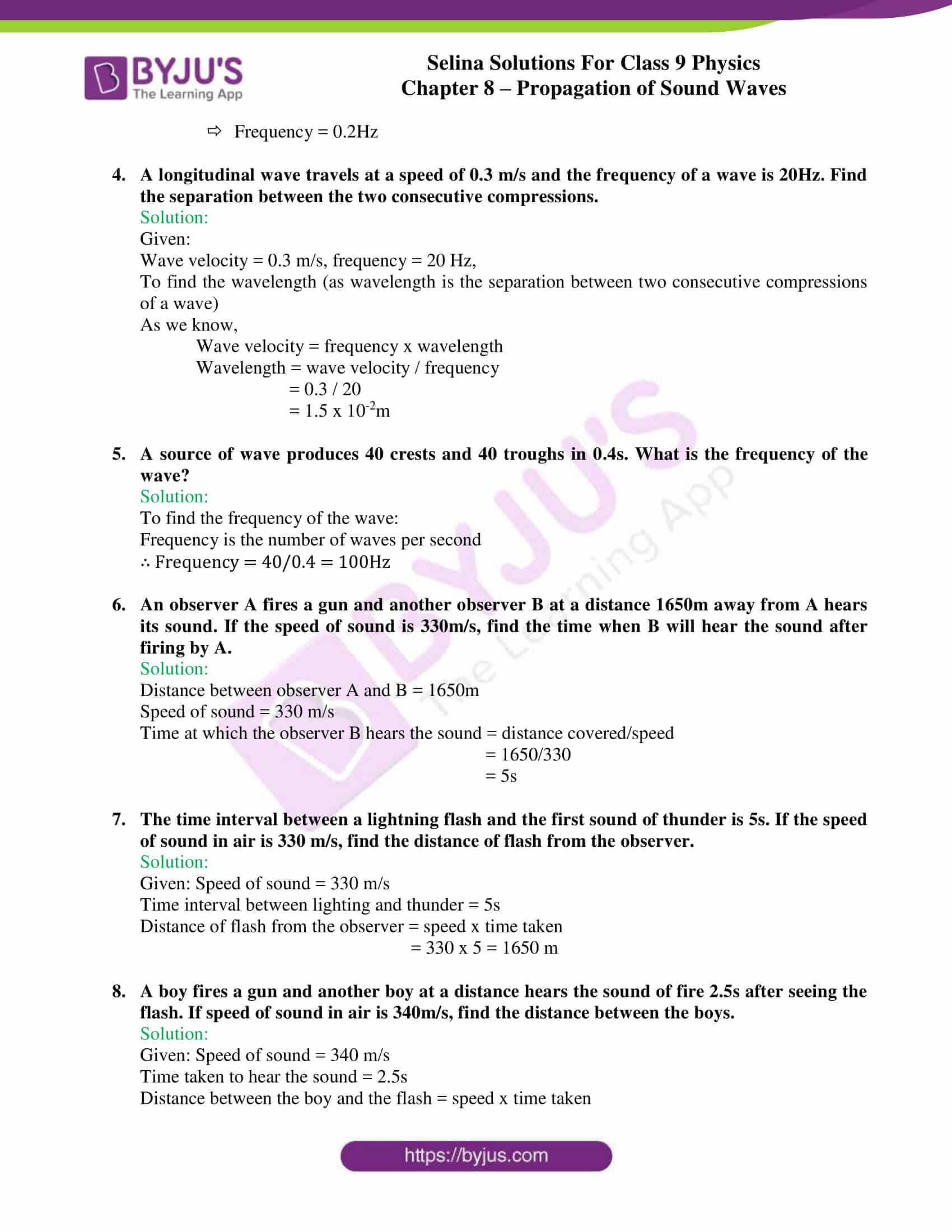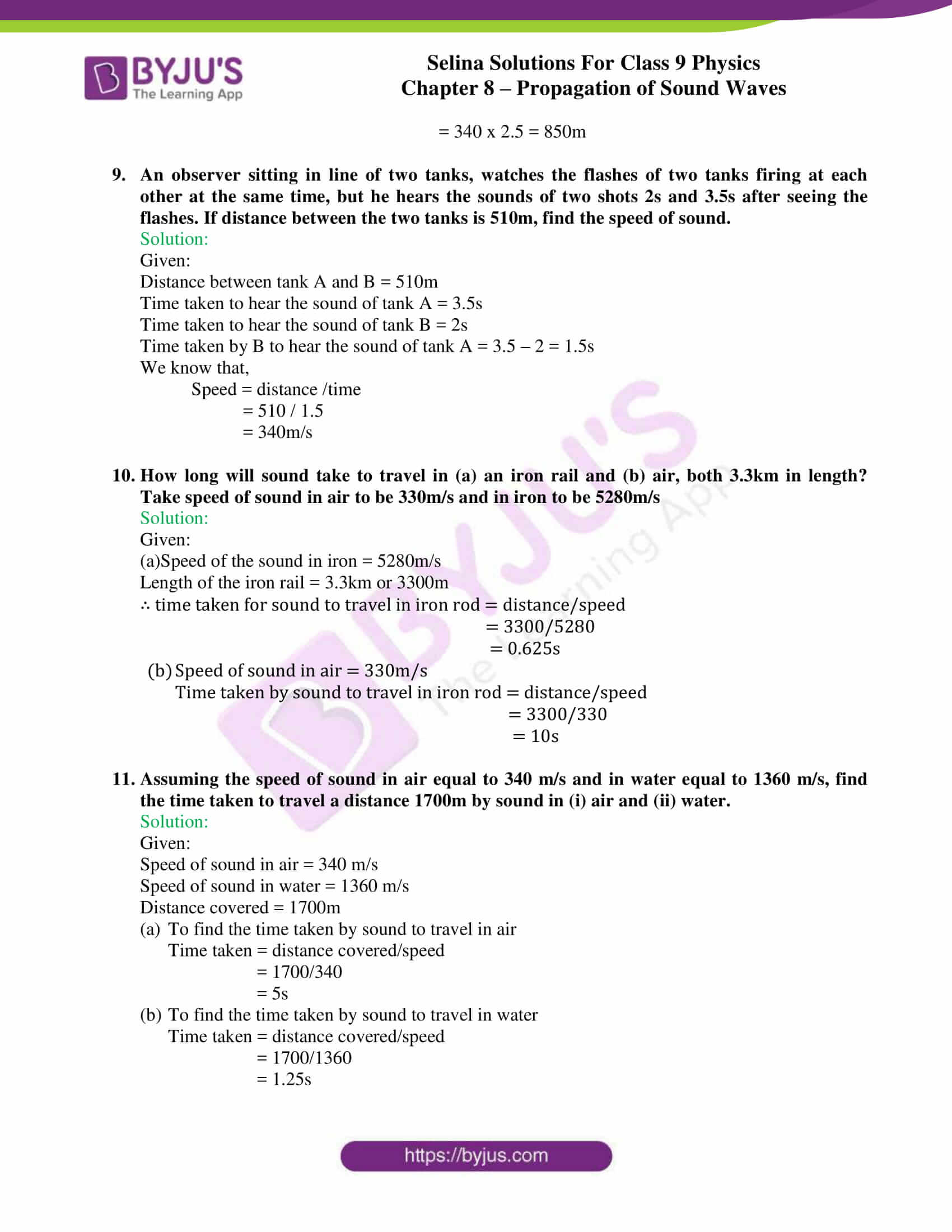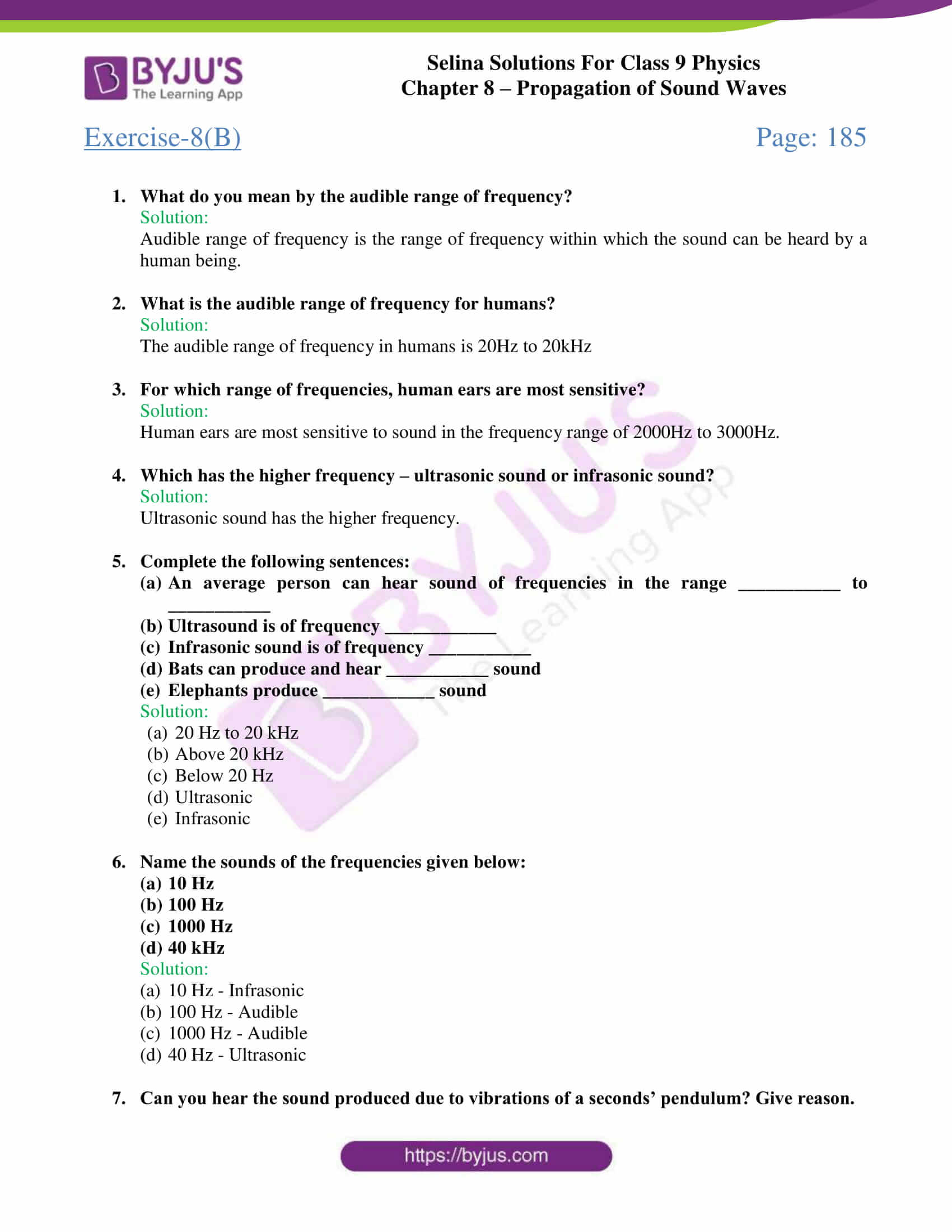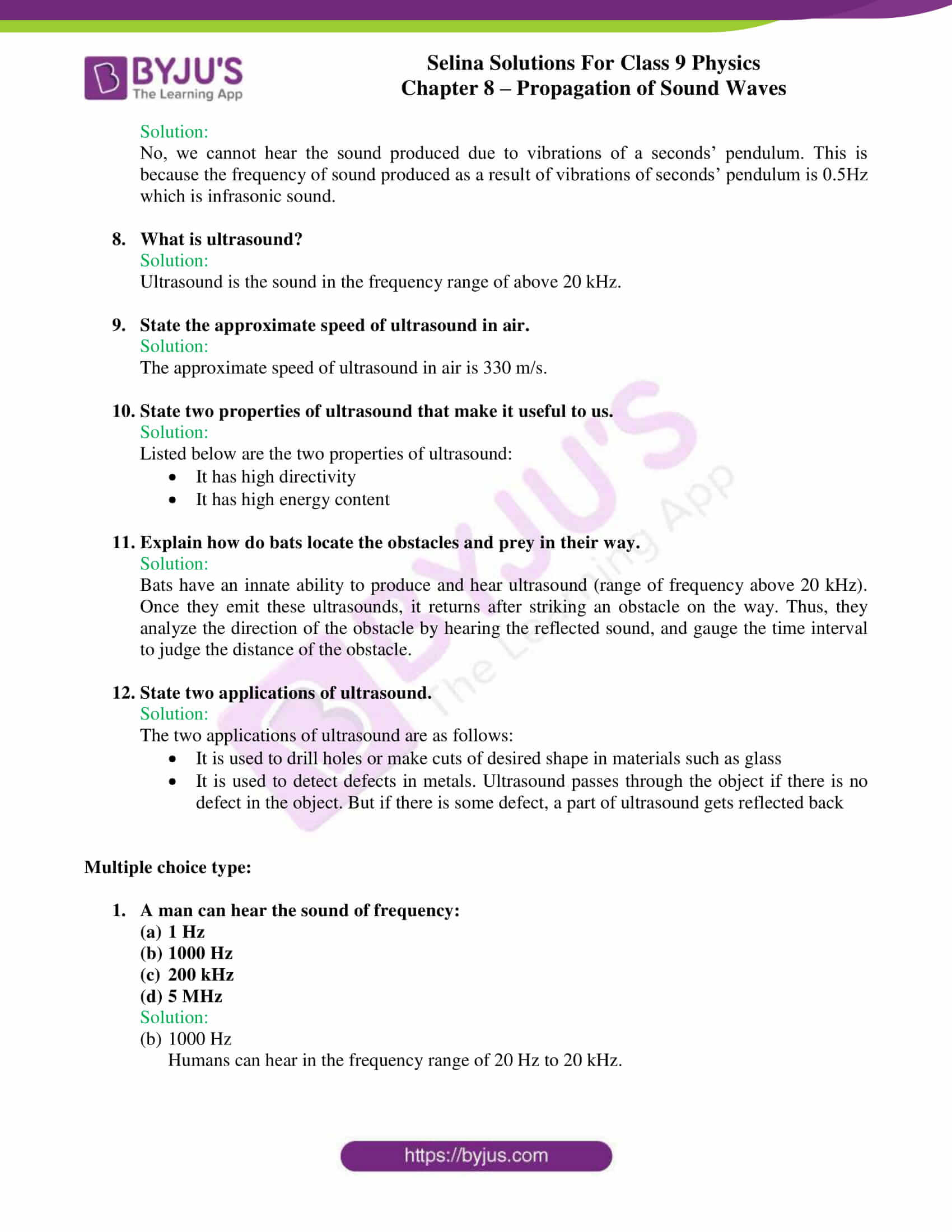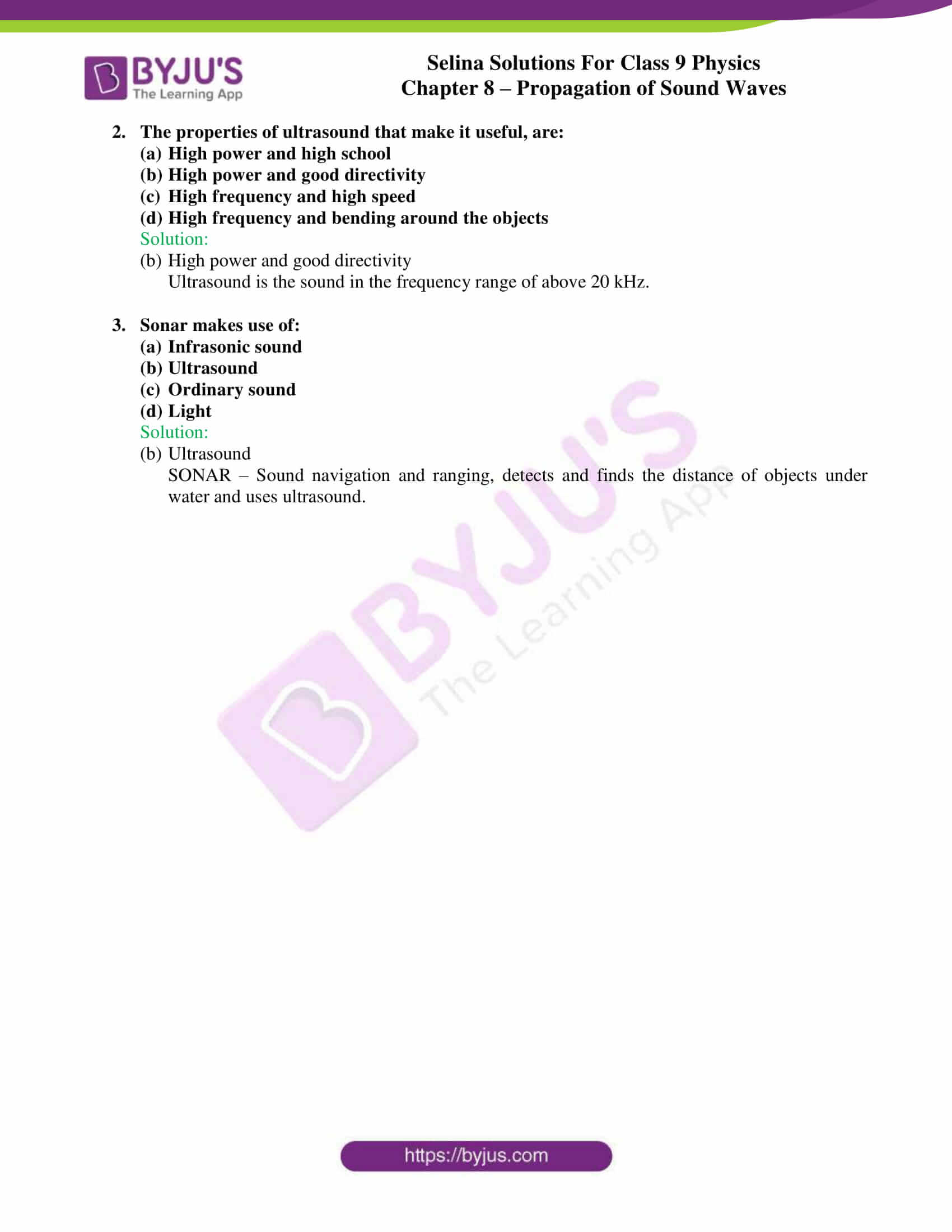## Selina Solutions for class 9 Physics Chapter 8 – Propagation of Sound waves

ICSE Class 9 Physics Propagation of sound waves is the 8th chapter covered in class 9 physics textbook. Sound is a vibration that typically arises from an object. Through this chapter, students understand how sound is produced from vibrations, for this, simple experiments have been demonstrated for the better understanding of students.

It also discusses how sound requires a material medium for its propagation through an experiment(bell jar experiment).

Some terms related to the topic such as amplitude, time period, frequency, wavelength, wave velocity, helps students understand the sound wave and enable them to solve numerical problems on the same. Displacement-time graphs, displacement-distance graph is the next thing students can look forward to.

Speed of sound varies in different media. Some factors affecting the speed of sound is also discussed here. Light travels faster than sound, a comparison with the speed of sound is made which helps students gauge many aspects related to it.

List of subtopics covered in Chapter 8 – Propagation of Sound waves:

 Number Subtopic 8.1 Sound and its production from vibrations 8.2 Sound propagation requires a material medium 8.3 Propagation of sound in a medium 8.4 Some terms related to wave motion 8.5 Relationship between the wavelength, wave velocity and frequency 8.6 Speed of sound in different media 8.7 Factors affecting the speed of sound in a gas 8.8 Factors not affecting the speed of sound in a gas 8.9 Comparison of speed of sound with the speed of light 8.10 Experimental determination of the speed of sound in air 8.11 Infrasonic, sonic and ultrasonic frequencies 8.12 Ultrasound and its applications

List of Exercise

 Name of the exercise Number of questions Page number Exercise-8A 51 182 Exercise-8B 15 185

## Selina Solutions for class 9 Physics Chapter 8 – Propagation of Sound waves

Propagation of sound waves covers important aspects in physics such as nature of sound waves, the requirement of sound waves to travel, propagation and speed in different media, comparison with the speed of light.

Some terms related to wave motion are amplitude, time period, frequency, wavelength, wave velocity etc. The chapter also discusses the relationship between the wavelength, wave velocity and frequency etc, which are fundamentals, that students come across often in this chapter. The various factors affecting the speed of sound in a gas is also discussed in this chapter.

The chapter also provides numerical problems towards the end of each exercise along with solved examples for practical application of theoretical concepts.

### Key Features of Selina Solutions for class 9 Physics 8 – Propagation of Sound waves

• Elaborate and easy to understand solutions
• Use of pointers and graphs wherever necessary
• Numerical problems are provided with accurate calculation and explanation
• Graphs and tables are provided wherever necessary
• Gives a better understanding of concepts

## Frequently Asked Questions on Propagation of Sound waves

Q1

### What causes sound?

Sound is caused when a body vibrates.

Q2

### What is sound? How is it produced?

Sound is a form of energy that produces the sensation of hearing in our ears. Sound is produced by vibrations.

Q3

### What is a transverse wave?

It is the wave in which the particles of the medium vibrate about their mean positions, in a direction that is perpendicular to the direction of wave propagation.

Q4

### Define the term wave velocity?

Wave velocity can be defined as the distance travelled by a wave in one second. It is also known as wave speed.

Q5

### How does the speed of sound in air vary with temperature?

The speed of sound in air varies. It increases by 0.61 m/s for each 1°C rise in temperature.

Q6

### What do you mean by the audible range of frequency?

Audible range of frequency is the range of frequency within which the sound can be heard by a human being.

Q7

### Did Ultrasonic sound has the higher frequency?

Yes, Ultrasonic sound has the higher frequency